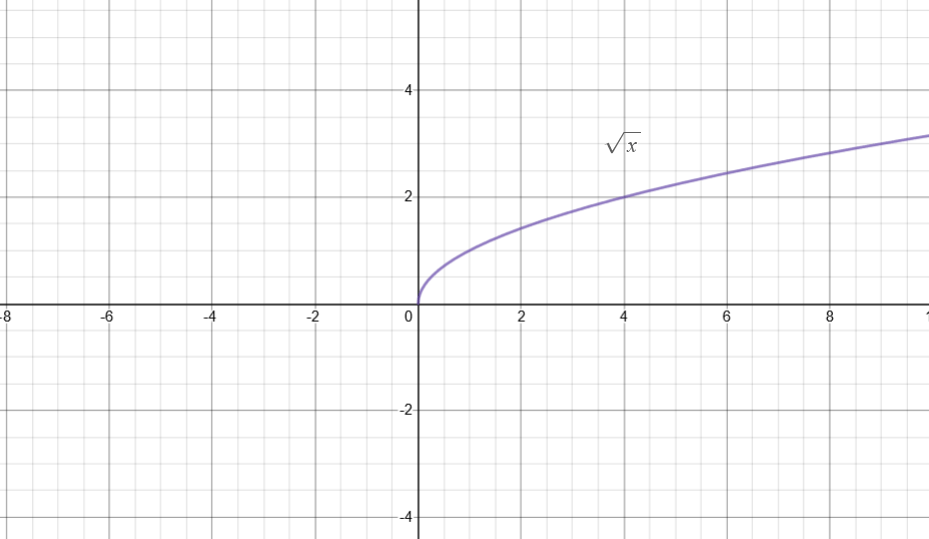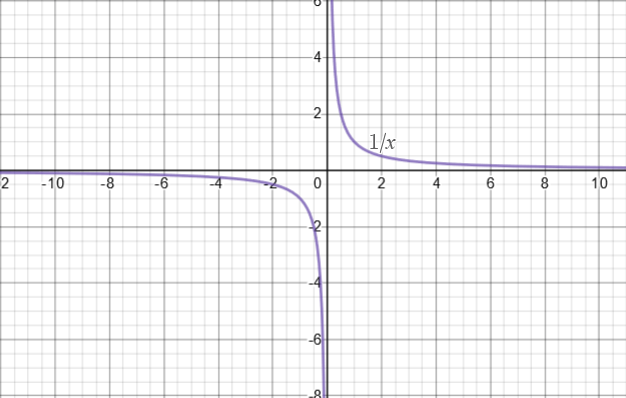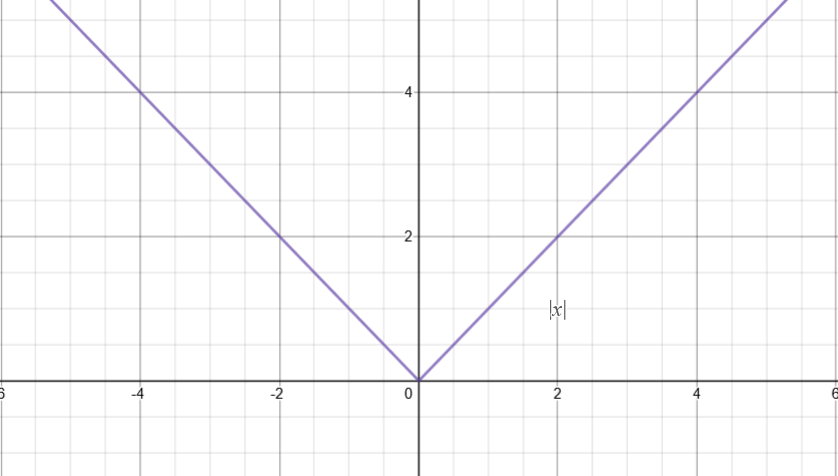# Domain and Range

Contents

You may recall what a function is and what it does. A function basically takes some values and outputs corresponding values after performing some operation on it. The operation can as simple as multiplying the input with 1 (basically doing nothing). In textbooks, a function is usually attributed as a mathematical machine that swallows a number, processes it (based on some rule) and spits out another number. Here we will discuss about those numbers (input and output), what they are called, their notation etc. in detail.

## Domain

You may recall that a function is represented in form of an expression that involves the use of an independent variable (usually represented with the symbol x). A function has to be passed some numbers as the values of the independent variable x. You should realize that some functions ‘break’ at some input values (or in other words, the rule that a function is based on cannot be applied to some numbers). For example, the function$f(x) = \frac{1}{x}$ is undefined when you plug in the number x = 0. Similarly, the function$f(x) = \sqrt{x}$ cannot output a real number for x < 0.

The lesson is that we should not be ignorant about on what input values a function is defined and on what input values it is not defined. The concept of domain arises as a result.

## Definition

The set of values of the independent variable for which a function f(x) is defined is called the domain of that function.

For example, the function$f(x) = \frac{1}{(x+1)^{2}}$ is defined for all values of  except -1 (because at x = -1, the function evaluates to$f(-1) = \frac{1}{(-1+1)^{2}}=\frac{1}{0}$ which is undefined and does not lie in set of Real numbers). Therefore, we say that the domain of$f(x) = \frac{1}{(x+1)^{2}}$ is the set of Real numbers except -1. Note that domain is a set, not a number.

Exercise: Find the domain of the function$f(x) = x^{2}$.

It is good practice in maths to express the domain of the function with its definition. In textbooks, you will find domains of functions written with their definition. For instance, you may find something like this,$f(x) = sin^{-1}(x),\,-1\leq x\leq 1$

The expression “$-1\leq x\leq 1$” basically represents the domain of the function. It says that the function$sin^{-1}$ is defined for the input values ranging from -1 to 1 (You do not have to worry about what the function$sin^{-1}$ is, at this point).

Interesting fact: A domain can further be constrained based on any limitations whatsoever. For example, suppose you define the speed of a car ‘v’ as some function of time ‘v’. It is practically non-sense to have negative time, therefore you can restrict the domain to only$t\geq 0$. Therefore, the domain represented with a function definition would look something like this.$v(t)=2t+1,\,t\geq 0$

Notation

There are a number of ways in which the domain of a function can be expressed. We explore them here.

1) Inequality notation: In this notation, we make use of the symbols$< ,\,> ,\,\leq,\,\geq$. For example, for the function$f(x) = \frac{1}{x^{'}}$, the domain can be represented as$-\infty

Or,$-\infty

where$\cup$ means “union of intervals”.

Or even,$-\infty

We can also have domains limited by finite numbers  and . We may have,

• a ≤x ≤b
• a < x ≤b
• a≤ x <b
• a< x <b

Similarly, any combination of infinity and finite numbers is also possible. Such as,

•$-\infty
•$a\leq x < \infty$

2) Set-builder Notation: This representation of the domain is characterized by ‘$\left \{ x | (expression of domain) \right \}$‘. The above defined function i.e.,$f(x) = \frac{1}{x}$ can be represented in set-builder notation as$\left \{ x | -\infty

It reads as “x,such that x is between -∞ and ∞, and x is not equal to 0”. The symbol “|” reads as “such that”. In other words, it says that the domain of$f(x) = \frac{1}{x}$ is the set containing x where x is so and so ….

3) Interval notation: This is yet another notation used to represent the domain. The interval notation makes use of the round and square brackets (,), [,], to replace the equality and inequality symbols. Round brackets are used to replace the <, > symbols, whereas square brackets are used to replace the ≤, ≥ symbols.

Taking the example of the function$f(x) = \frac{1}{x}$ yet again, the domain can be represented in interval notation as$(-\infty, 0)\cup (0,\infty)$

Or,$(-\infty, \infty), \, x\neq 0$

The round bracket with 0 represents that 0 is not included. If zero were included, we would have used the square bracket. For example, the domain of the function$f(x) = \sqrt{x}$ is represented in interval notation as

[0,∞)

Or with the function,$f(x) = \sqrt{x},\, x \,\epsilon \,[0,\infty)$

Exercise: Find the domain of the function$f(x) = \frac{1}{x^{2}-4}$ and represent it using all of the three notations discussed above.

## How to find the Domain of a function?

Two methods will be discussed in this article. First is the analytical method.

If you are given the expression of a function, then you should try to find where function is not defined. Once you find that, you just have to exclude that region of input values. An easy way to do that is to see if there is any fraction in the expression where the independent variable x lies in the denominator. If that is the case, the domain of the function will exclude all those values of x for which the denominator equates to 0. For example, if$f(x) = \frac{2x}{x-5} + \frac{3}{x+1}$, you should immediately realize that the denominator becomes 0 at x = -1  and x = 5 and nowhere else. Thus, the domain of the function becomes the set of real numbers excluding the numbers -1 and 5. It is also possible that the function includes square root. If a square root appears, then you have to make sure that the inside of it does not become negative. For example, if$f(x) = \sqrt{x-4}$, then it can be noticed that the expression (x – 4) becomes negative for x < 4. Therefore, the domain of the function turns out to be [4,∞) i.e., the function is defined for x equal to and greater than 4. Notice that you will always find the infinity symbol  with a round bracket.

The second method will be discussed later when we will look at the graph of a function.

## Domain of a Piecewise function

The domain of a piecewise function is mentioned in its expression. For example, for the piecewise function$f(x) = \left\{\begin{matrix}-x, & x <0\\ x,& x\geq 0\end{matrix}\right.$

But how to deal with two separate intervals? It is surprisingly easy. Just take the union of the intervals. Therefore, the domain of this function is the union of the set of negative real numbers and set of positive real numbers (including zero). Thus, the domain of this piecewise function is$\mathbb{R}$ i.e., the set of real numbers. The domain of the piecewise function,$f(x) = \left\{\begin{matrix}1, & 0\leq x<10\\ x, & 10\leq x<20\\ 0,& x\geq 20\end{matrix}\right.$

is the union of three intervals i.e., [0,10)∪[10,20)∪[20,∞). In other words, the set of all positive real numbers including 0.

The domain of the following piecewise function,$f(x) = \left\{\begin{matrix}x^{2},& 31.23\leq x<40\\ \sqrt{x},& 100

is the union of two intervals, i.e., [31.23,40)∪(100,500). Note the use of brackets. It should also be noted that the form in which the domain is represented here with the definition of the piecewise function is the Inequality form.

Exercise: Express the domain of the following piecewise functions in the Set-builder notation and the Interval notation.$g(x) = \left\{\begin{matrix}\frac{1}{x^{2}}, & x <0\\ \frac{1}{x},& x> 0\end{matrix}\right.$$h(x) = \left\{\begin{matrix}1, & x =0\\ -1,& x\neq 0\end{matrix}\right.$

## Domain of function in form of a Set of ordered pairs

As you may recall that a function maps input values to output values. This relation can be defined in several possible ways (equation is one of the possible ways of representation!). It is also possible that a function is conveyed in the form of a set of ordered pairs of numbers. For example,

{(0,1),(1,2),(2,3),(3,4)}

is a set of four ordered pairs (a, b). The first number in each of the ordered pairs represent the input whereas the second number represents the output. Therefore, we can say that the domain of this relation (a function actually. Can you see why is it a function?) is {0,1,2,3}. This finite set can also be represented in the form of three notations that we have studied earlier.

Inequality notation: 0 ≤ x ≤ 3, x ϵ$\mathbb{Z}^{+}$

Set-builder notation: {x | x ϵ$\mathbb{Z}^{+}$, x ≥ 0 ˄ x ≤ 3}

Interval notation:[0,3],x ϵ$\mathbb{Z}^{+}$

There are several things to notice here. The symbol ‘$\mathbb{Z}^{+}$’ represents the set of positive integers (It is a must to mention that x is an element of$\mathbb{Z}^{+}$ so that one may know that only the whole numbers should be considered. Otherwise, if nothing is mentioned that it is automatically considered that the numbers belong to the set of Real numbers). Second, the symbol “˄” means “and”. This symbol is frequently used in set-builder notation.

Exercise: Find the domain of the relation represented in the form of set of ordered pairs as {(1,11), (2,21), (3,31), (-1,-9), (-2,-19)}. Also comment whether this relation represents a function or not.

## Range

A function outputs a set of objects or numbers based on the input numbers or objects (from the set of domain). This set is called the Range. In simple words, a function maps the set of Domain into the set of Range.

In textbooks, you will find the set of domain represented by the letter X while the set of range represented by the letter Y.

## Definition

The set of values of the dependent variable (y = f(x)) that are, in essence, the outputs of a function f(x), is called the range of that function.

For example, the range of the function$f(x) = \frac{1}{(x+1)^{2}}$ is set of all positive real numbers except 0. Therefore, its range can be written as “$y \, \epsilon \,\mathbb{R}^{+} -0$”. Observe that the above function maps the real numbers between -1 and 0 to the real numbers from 1 to ∞! The range between only 0 and 1 is map of the domain from 0 to ∞ (i.e., almost all of the domain is mapped to real numbers between 0 and 1).
It should be noted that Range is basically an Image of the Domain under the transformation f(x). For two different functions$f_{1}(x)$ and$f_{2}(x)$ with same domain, their respective ranges may or may not be different. For example, the domain and range of both$f_{1}(x) = x^{3}$ and$f_{2}(x) = x^{5}$ are same whereas, the respective ranges of$f_{1}(x)$ and$f_{3}(x) = x^{2}$ for the same set of domain are different.

Exercise: Find the range of the function$f(x) = x^{2}$.

## How to find the range of a function?

Two methods are discussed in this article. One analytical method and other graphical.

In the analytical method, we try to imagine the behaviour of the function near the extremes in the domain (e.g., negative and positive infinites) and near the special points (e.g., 0 for$f(x) = \frac{1}{x}$).
We also look for the terms with even and odd exponents. Terms with even exponents always evaluate to positive numbers whereas the terms with odd exponents evaluate to both negative and positive numbers.

Often there are special functions such as exponential functions, trigonometric functions, logarithmic functions etc. that we should be careful about.

Notation

The same notations that we defined for the domain can be used to represent the range of a function. Thus, only an exercise is presented.

Exercise: Find the range of the function$f(x) = \frac{1}{x^{2}-4}$ and represent it using all the three notations i.e., Inequality notation, Set-builder notation, Interval notation. Do not forget to replace the symbol x with y (used to represent the output of a function) in the notations.

## Range of a Piecewise function

Sometimes you may have to deal with piecewise functions. A piecewise function can be thought of as union of two or more separate functions (with their separate domains). So, nothing special needs to be done. Just identify the range of each “separate function” and take the union.

Let us revisit the examples of the piecewise functions and find their respective ranges.

For the following function, it can be seen that the output is always positive (equal to the absolute magnitude of the input real number).$f(x) = \left\{\begin{matrix}-x, & x<0 \\ x,& x\geq 0\end{matrix}\right.$

Therefore, the range is (0,∞)∪[0,∞)=[0,∞).

Remember, always work systematically. Find the range of each part of function separately. After then, take their union. Deal with a piecewise function, piece wise!

Next, we have the following function.$f(x) = \left\{\begin{matrix}1, & 0\leq x<10 \\ x, & 10\leq x<20\\0,& x\geq 20\end{matrix}\right.$

Range for the first part is  (it outputs 1 for all values of input in domain), range for the second part is [10,20) (Note the use of brackets) and the range for the third part is . The overall range for the function f(x) is ∪[10,20)∪.

Similarly, for the next function$f(x) = \left\{\begin{matrix}x^{2}, & 31.23\leq x<40 \\ \sqrt{x},& 100

The range for first part is [975.3129, 1600) i.e., set of square of domain values. The range for the second part is (10, √500). The overall range of the function is (10, √500)∪[975.3129, 1600).

Always be vigilant about the use of round versus square brackets while writing the domain or range of a function. Now, it is time for exercise.

Exercise: Express the range of the following piecewise functions in the Set-builder notation and the Interval notation.$g(x) = \left\{\begin{matrix}\frac{1}{x^{2}}, & x <0\\ \frac{1}{x},& x> 0\end{matrix}\right.$$h(x) = \left\{\begin{matrix}1, & x =0\\ -1,& x\neq 0\end{matrix}\right.$

## Domain and Range from Graph

Now we discuss how to visualize domain and range of a function and importantly, find the domain and range from the graph of a function. As a matter of fact, you will observe that it is rather a piece-of-cake to identify the domain and range from the graph of a function. By looking at the graph of a function, the domain and range will be instantly realized.

Let us start. First, remember that the domain is always along the horizontal axis i.e., x-axis, whereas the range is always along the vertical axis i.e., y-axis on a graph.

Caution: Domain is along the x-axis, not necessarily all the values on x-axis. Similarly, Range is along the y-axis, not necessarily all the values on y-axis.

Domain on the graph of a function is that region of x-axis for which a part of the curve of the function exists. The region of x-axis for which you observe the plot does not exist is excluded from the domain. For example, see the graph of f(x) = √x below.It can be noticed that the curve does not exist for left half x-axis. Therefore, the domain of f(x) = √x is the set of positive real numbers only. The range of this function is also the set of positive real numbers only (The curve does not exist for negative portion of y-axis).

It is possible that the curve may not exist only for a single value on x-axis. For example, see the graph of$f(x) = \frac{1}{x}$ below.The function blows to infinity at x = 0. Therefore, the domain of the above function is set of real numbers excluding x = 0. Similarly, along the y-axis, the curve never crosses y = 0, although it varies from negative to positive infinity. Therefore, the range of the function is also the set of real numbers excluding y = 0.

Let us take a look at an interesting example.This is graph of a trigonometric function cos cos x . Note that the curve exists along the total length of the x-axis. The domain of cos cos x , therefore, is the set of real numbers.

What about the range? Is it also the set of real numbers? Observe the curve and the y-axis. The curve only exists between the y values of -1 and 1. Therefore, the range of cos⁡(x) is [-1, 1] i.e., -1 ≤y ≤1.

Exercise: Find the domain and range of the function graphed below.Sources: